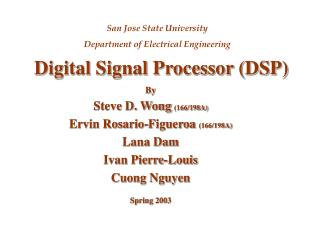DownloadDownload PresentationDigital Signal Processor (DSP)

Digital Signal Processor (DSP)

Download PresentationDigital Signal Processor (DSP)

- - - - - - - - - - - - - - - - - - - - - - - - - - - E N D - - - - - - - - - - - - - - - - - - - - - - - - - - -
Presentation Transcript

1. By Steve D. Wong (166/198A) Ervin Rosario-Figueroa (166/198A) Lana Dam Ivan Pierre-Louis Cuong Nguyen Spring 2003 Digital Signal Processor (DSP) San Jose State University Department of Electrical Engineering

2. Outline • Introduction • Specification • Project Purpose

3. Introduction • Design of a 4-Bit Digital Signal Processor (DSP) using CMOS Logic. • DSP is composed of a Multiplier, D Flip Flop and a Subtractor. • Up/Down Counter will be used as a test vector for the system.

4. Specifications • Functional Specification 4-Bit Multiplier 4-Bit Full Subtractor D Flip Flop 4-Bit Up/Down Counter • Technical Specifications Design Wn & Wp = 3 mm Power <= 0.25Watt Clock Frequency << 200 MHz VDD = 5 Volts

5. Schematic (4-Bit Multiplier) Schematic Layout

6. Test Bench (4-Bit Multiplier)

7. Simulation (4-Bit Multiplier)

8. Layout (4-Bit Multiplier)

9. Extract (4-Bit Multiplier)

10. LVS (4-Bit Multiplier)

11. Schematic (4-Bit Subtractor)

12. Test Bench (4-Bit Subtractor)

13. Simulation (4-Bit Subtractor)

14. Layout (4-Bit Subtractor)

15. Extract (4-Bit Subtractor)

16. LVS (4-Bit Subtractor)

17. Schematic (D Flip Flop)

18. Test Bench (D Flip-Flop)

19. Simulation (D Flip Flop)

20. Layout (D Flip-Flop)

21. Extract (D Flip Flop)

22. LVS (D Flip Flop)

23. Schematic (Up/Down Counter)

24. Test Bench (Up/Down Counter)

25. Simulation (Up/Down Counter)

26. Layout (Up/Down Counter)

27. Extract (Up/Down Counter)

28. LVS (Up/Down Counter)